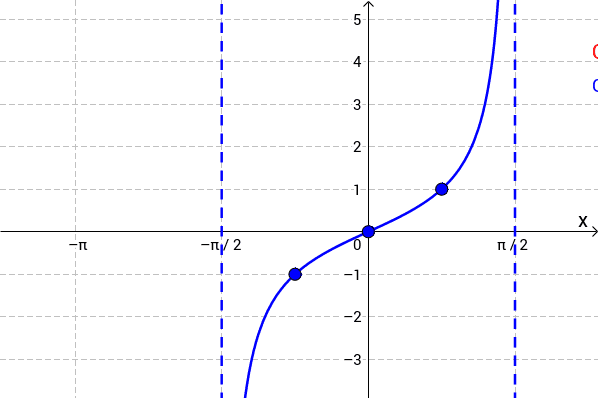# Tangent free online calculator

Online trigonometric tangent calculator.

## Tangent calculator

* Use e for scientific notation. E.g: 5e3, 4e-8, 1.45e12

## Tangent definition

In a right triangle ABC the tangent of α, tan(α) is defined as the ratio between the side opposite to angle α and the side adjacent to the angle α:

tan α = a / b

#### Example

a = 3"

b = 4"

tan α = a / b = 3 / 4 = 0.75

## Graph of tangent## Tangent rules

Rule nameRule
Symmetry

tan(-θ) = -tan θ

Symmetrytan(90°- θ) = cot θ
tan θ = sin θ / cos θ
tan θ = 1 / cot θ
Double angletan 2θ = 2 tan θ / (1 - tan2 θ)
Angles sumtan(α+β) = (tan α + tan β) / (1 - tan α tan β)
Angles differencetan(α-β) = (tan α - tan β) / (1 + tan α tan β)
Derivativetan' x = 1 / cos2(x)
Integral∫ tan x dx = - ln |cos x| + C
Euler's formulatan x = (eix - e-ix) / (eix + e-ix)

## Inverse tangent function

The arctangent of x is defined as the inverse tangent function of x when x is real (x∈ℝ).

When the tangent of y is equal to x:

tan y = x

Then the arctangent of x is equal to the inverse tangent function of x, which is equal to y:

arctan = tan-1 x = y

#### Example

arctan 1 = tan-1 1 = π/4 rad = 45°

See: Arctan function

## Tangent table

x

x

(°)

tan(x)
-π/2-90°-∞
-1.2490-71.565°-3
-1.1071-63.435°-2
-π/3-60°-√3
-π/4-45°-1
-π/6-30°-1/√3
-0.4636-26.565°-0.5
00
0.463626.565°0.5
π/630°1/√3
π/445°1
π/360°3
1.107163.435°2
1.249071.565°3
π/290°

Currently, we have around 5612 calculators, conversion tables and usefull online tools and software features for students, teaching and teachers, designers and simply for everyone.